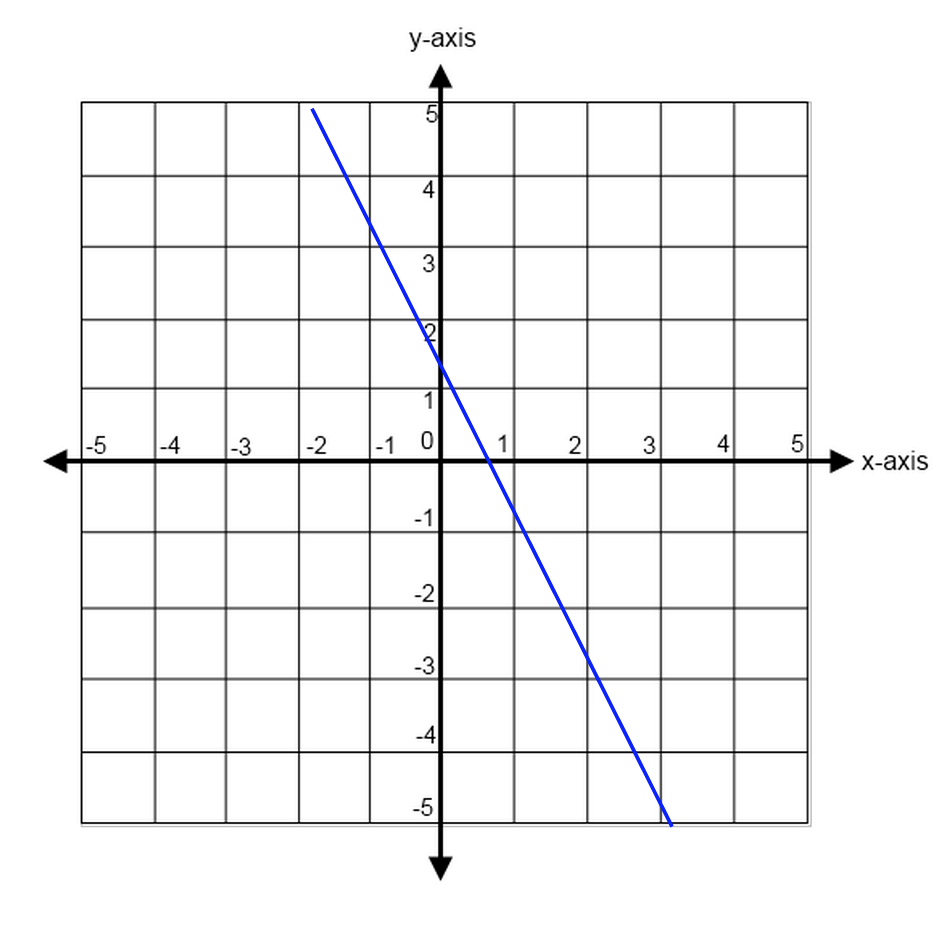Table
Graph
Equation
Two Points
Mixed

### 1003

### 1004/3

x + y =-15

m=-1

### 100

(-4, 2) and (5,6)

4/9

(2,2) & (0,12)

m=-5

### 2004

### 200-2

2y=-1x-8

m=-1/2

(5,-5) and (7,3)

4

### 2002

### 300-2

### 3003/4

4x - 2y = 12

m=2

### 300

(2,1) and (3,-10)

-11

### 300

(2,1) and (3,-10)

-11

### 4002

### 400-3/2

3x - 2y = 9

m=3/2

(9,8) and (5,1)

7/4

y + 8 = 12

m=0

### 5005

### 500-2/5

-2x + y=1

m=2

### 500

(-8,-5) and (-7,-4)

1

y - 4=-3(x-3)

m=-3